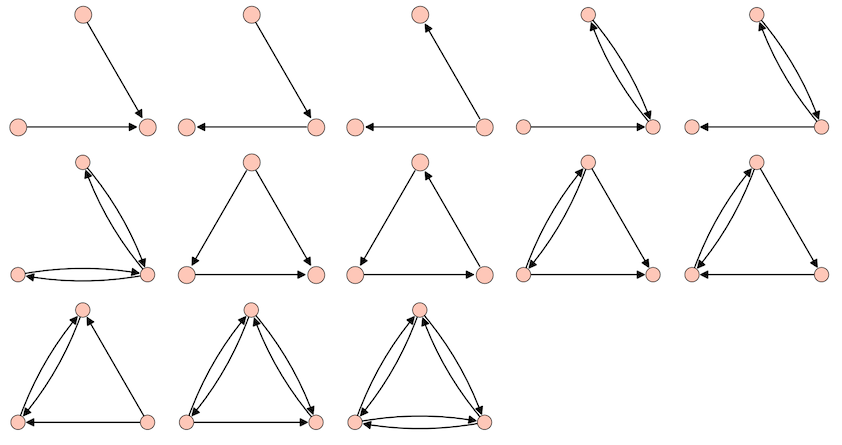# Revision history [back]

To get all the connected directed graphs on 3 vertices (no multiple directed edges, no loops) up to isomorphism, one can use graphs.nauty_geng and digraphs.nauty_directg as follows:

sage: n = 3

sage: graphs_n = [g for g in graphs.nauty_geng(f"-c {n}")]
sage: digraphs_n = list(digraphs.nauty_directg(graphs_n))

sage: len(digraphs_n)
13

sage: opt = dict(layout='circular', vertex_size=600, vertex_labels=False)
sage: opt = dict(xmin=-1, xmax=1, ymin=-0.7, ymax=1.1, **opt)
sage: G = graphics_array([g.plot(**opt) for g in digraphs_n], ncols=5)

sage: G.show(figsize=(17, 9))
Launched png viewer for Graphics Array of size 3 x 5